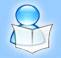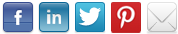A Collection of Informative and Interesting Articles
 HOME WANT AN ACCOUNT? SUBMIT ARTICLES TOP AUTHORS Debt Collections (Advt.)

# Explaining Unified Field Theory

BY: Bob Ticer | Category: Others | Submitted: 2017-05-12 06:40:41Article Summary: "Einstein spent many of his years attempting to formulate a unified field theory. I believe the answer lies with more modification of Newton's theory of gravity in that sized is relative..."Einstein attempted, for many years of his life, to formulate a unified field theory. A possible solution pertains to overcoming false assumptions. Einstein challenged Newton's assumptions of absolute space and absolute time in replacing them with relative space-time. Another false assumption could relate to the relativity of size. It involves modifications of both Newton's inverse-square-law of gravity and Einstein's space-time curvature of general relativity.
Adrien Maurice Dirac once speculated on the nature of the Hubble Constant H in relation to gravity. He noted they could be mathematically related to the present time, but that modification of theory is needed due to the value of H decreasing as the universe expands. He realized H could remain constant if Newton's constant of gravity G decreases as well. However, included in the formulation of an expanding universe is a Cosmological Principle whereby observers perceive themselves as relatively at the center of the universe. This perception is possible if the path of light curves back at the edge of the universe, but expansion of the universe nullifies space-curvature of gravity even without a decrease in G.
Suppose gravity is part of electromagnetism in that it relatively decreases with less mass density. Light speed relatively decreases in a gravitational field. It thus becomes faster if gravity decreases. In view of the Cosmological Principle, assume light speed becomes faster along with clock rates such that no change is perceived. With this nullification, the universe could be perceived as static, as neither expanding nor shrinking, as Einstein originally assumed. However, this condition constitutes a modification of theory. It assumes size is relative.
The modification is not without precedence. Horace Babcock, as early as 1939, noted a discrepancy in the rotational speed of the Andromeda galaxy. In the 1960s and 1970s, a general study of galaxies under the guidance of Vera Rubin found rotational speeds away from galactic centers do not decrease according to Newtonian theory. (Dark matter is now assumed to exist because of the findings.) In 1983, Mordehai Milgrom proposed a modification of Newton's inverse-square-law. In 2004, Jacob Bernstein offered a relativistic modification of space-time curvature.
According to Newton, if mass and its radius are both double, rotational speed is the same. However, the mass density is one-fourth. This less mass density might allow greater light speed and faster clock action because of less gravitational interaction per space. Consistent with this assumption is that the average density of the universe per average nuclear density of the hydrogen atom appears to equal the ratio of gravitational force to electrostatic force between the proton and the electron.
A probability condition of Quantum Electrodynamics (QED) is directly related to the Planck Constant h, having parameters mvr for mass, velocity and radius. If the event horizon of a black hole is at radius R for the limit of total changes in speeds being light speed c, and if R is substituted for r, and Dr/137 is substituted for v, as the difference in speed at distance r/137, then the value of h does not change. It does not change because v/c = 1/137 for a shorter distance of quicker light action, and also because of R being the distance-range-limit of the different speeds, whereby mDRr/137 = mvr. General relativity thus complies with the probability condition of QED.
Theories equate. The math only needs to be interpreted in consistent manner along with the evidence.
Galaxies are not themselves black holes, but the universe as a whole compares as one. It might not be a mere coincidence that if 2r is the diameter of the nuclear radius, and R is the radius of the universe, then 2r/R appears to equal the ratio of gravitational to electrostatic forces between the electron and proton. Moreover, H2r/c, as the difference in either the speed or energy value of H at distance 2r per light speed, also equals the ratio of gravitational to electrostatic forces of the electron and proton.
What is now needed is another Einstein who can formulate these results into consistent theory.

After four years in the USAF i took a philosophy class and came up with an idea for explaining gravity. After retiring in 1999, I began writing and research. I recently published two books: Explaining Gravity Simple Consistent And Complete and The Alien Stranger.

 Comment Comment By Comment Date

•   Live For Others

•   What is Forex Trading?

•   House Interior Designs and Colors According to Feng Shui

•   Positive Attitude is Key to Success

Latest Articles in "Others" category:
•   Understanding of the World

•   The Run, the Old Man and Me !

•   Ayahuasca ....here I Come !

•   A General Disussion

•   A Narrative on Animals and Birds

•   A Big Bang Debate

•   Another Day

Important Disclaimer: All articles on this website are for general information only and is not a professional or experts advice. We do not own any responsibility for correctness or authenticity of the information presented in this article, or any loss or injury resulting from it. We do not endorse these articles, we are neither affiliated with the authors of these articles nor responsible for their content. Please see our disclaimer section for complete terms.Copyright © 2010 saching.com - Do not copy articles from this website.
| Home | Disclaimer | Xhtml |